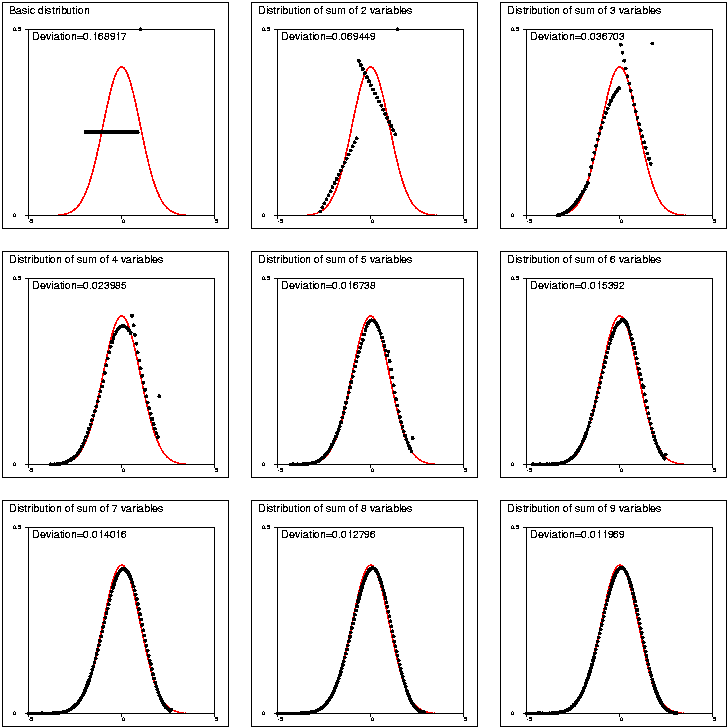# Environment for creative processing of text and numerical data## Central limit theorem

S.Mustonen (2003)### Edit field:

```   1 *
2 *Central limit theorem
3 *
4 *Sucro SUMDISTR shows how the sum of independent random variables
5 *tends to the normal distribution when the number of variables grows.
6 *SUMDISTR works in the special case where the variables have the same
7 *discrete distribution given by the user.
8 *SUMDISTR displays density functions of sums on the screen automatically
9 *step by step and compares them to the normal distribution.
10 *It also computes a deviation from the normal distribution according to
11 *the Kolmogorov-Smirnov test statistics.
12 *
13 *Here only a collection of pictures is shown by letting the sucro
14 *save the partial graphs in a PostScript file.
15 *
16 *The sum distribution may have very interesting transient forms
17 *depending on the basic distribution before the ultimate 'normalization'.
18 *In this example we have a discrete uniform distribution with values
19 *0,1,2,...,19, but the probability of 19 is ten-fold when compared to
20 *others. Thus there is a peak on the right side of the distribution.
21 *
22 *It is sufficient to give values proportional to actual probabilities.
23 *`MAT Q1=CON(20,1)`               / Definition of probabilities as a vector
24 *`MAT Q1(20,1)=10`                / Setting the peak
25 *`/SUMDISTR Q1`  / NMAX=9 PS=SUM  / Running the demo and saving the graphs
26 *
27 *Overlaying density function of normal distribution (SUM0, also plotted
28 *by SUMDISTR) and the densities of sums:
29 *`EPS JOIN S1,SUM0,SUM1`
30 *`EPS JOIN S2,SUM0,SUM2`
31 *`EPS JOIN S3,SUM0,SUM3`
32 *`EPS JOIN S4,SUM0,SUM4`
33 *`EPS JOIN S5,SUM0,SUM5`
34 *`EPS JOIN S6,SUM0,SUM6`
35 *`EPS JOIN S7,SUM0,SUM7`
36 *`EPS JOIN S8,SUM0,SUM8`
37 *`EPS JOIN S9,SUM0,SUM9`
38 *
39 *Combining the partial graphs and setting a 3 x 3 array of pictures
40 *as a PostScript file ALL.PS:
41 *`EPS JOIN ALL,A1,A2,A3,A4,A5,A6,A7,A8,A9`
42 *A1=S1,0000,1100,0.333,0.333
43 *A2=S2,0550,1100,0.333,0.333
44 *A3=S3,1100,1100,0.333,0.333
45 *A4=S4,0000,0550,0.333,0.333
46 *A5=S5,0550,0550,0.333,0.333
47 *A6=S6,1100,0550,0.333,0.333
48 *A7=S7,0000,0000,0.333,0.333
49 *A8=S8,0550,0000,0.333,0.333
50 *A9=S9,1100,0000,0.333,0.333
51 *A9=S9,1100,0000,0.333,0.333
52 *

```